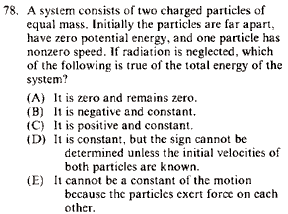GR 8677927796770177 | # Login | Register

GR8677 #78
Problem
 GREPhysics.NET Official Solution Alternate SolutionsMechanics}Conservation of energy

There isn't much to say about this problem other than the fact that it's a conceptual conservation of energy problem. Particle 1 moves at velocity towards particle 2, initially at rest. EM-potential energy increases as they get closer and closer together---but, energy should still be conserved since no energy is radiated. The potential energy increase comes from the initial kinetic energy of particle 1. Take choice (C). (The other choices are all either too weird or just plain bogus.)Alternate Solutions
 Saint_Oliver2013-09-13 20:08:00 since Therefore the total energy is greater than 0, which leaves only choice (C).Reply to this commentSaint_Oliver
2013-09-13 20:08:00

since

Therefore the total energy is greater than 0, which leaves only choice (C).thebigshow500
2008-10-14 01:41:19
So what will happen if radiation involves? I can't get a concise answer from this case. Anyone care to explain?
 Poop Loops2008-11-05 21:21:34 Makes things a lot harder. Charged particles radiate when they accelerate or decelerate. Things with mass accelerate or decelerate under a force. So they'd be pushing each other away and radiating, etc. So it's weird.grae313
2007-10-07 18:34:19
Yosun, you assume the particle with a velocity is moving towards the stationary particle, but what if it was moving away from the particle? The answer must be valid for both cases (moving towards the particle, or away), and in the case where it moves away, potential energy will always be zero and the total energy will just be given by the positive kinetic energy. Thus, C is clearly the answer.
 511mev2009-11-05 21:57:33 I know this is an old comment, but i'd still like to clarify: the potential energy is only zero at time zero, but since the coulomb potential is conservative, then T + V = a constant (of unspecified sign, since we don't know the signs of the charges).
 llama2013-10-16 18:19:15 T+V is a positive constant, since initially V=0 (as stated in the question) and T>0 (since .5*mv^2>0)dbiggerstaff
2005-11-10 00:13:03
There\'s a simpler way to look at it: the problem says that each particle initially has zero potential energy, but that one particle has a velocity relative to the other, which implies that it will have some kinetic energy in this reference frame. This means the total initial energy is T+U = (something)+0 = (something positive.) Since there\'s no radiation, energy will be conserved, and the total energy will remain (something positive.) Thus, the correct answer is (c).
 nitin2006-11-16 13:36:49 Thumbs up mate!
 abc1112009-10-02 02:54:55 can anyone explain... the sign cannot be determined unless intial velocity is known.....i am thinking...T is only thing that contains velocity here...but it will absorb minus sign because 1/2 m v^2 has a square term...
 kaic2013-10-09 13:37:53 It's highly unlikely that the particles would have negative energy, don't you think? While negative energy is not forbidden, and has been demonstrated to be possible, it's unlikely to show up on the GRE unless it is explicitly included as part of a question. As you said, the sign of the initial velocity does not matter because it is squared in the kinetic energy, so T is positive, and U is 0, so total energy E = T + V is positive.LaTeX syntax supported through dollar sign wrappers $, ex.,$\alpha^2_0$produces . type this... to get...$\int_0^\infty\partial\Rightarrow\ddot{x},\dot{x}\sqrt{z}\langle my \rangle\left( abacadabra \right)_{me}\vec{E}\frac{a}{b}\$